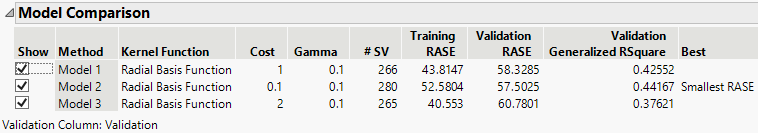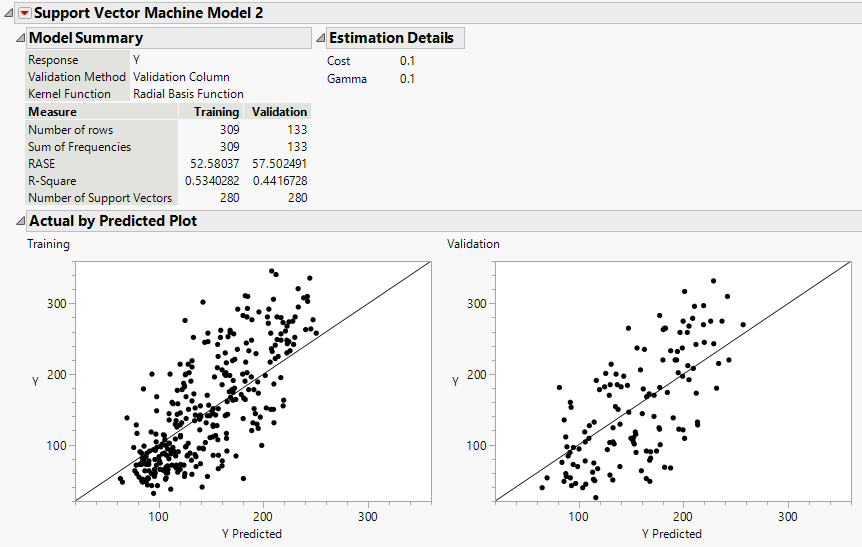Predictive and Specialized Modeling > Support Vector Machines > Additional Example of the SVM Platform
Publication date: 11/10/2021

# Additional Example of the SVM Platform

## Example of Support Vector Regression for Continuous Response

Support vector regression (SVR) models use the SVM algorithm to predict continuous response data. This example uses the same medical data on diabetic patients that was used in Example of Support Vector Machines. Instead of a binary measure of diabetes disease progression, you now have a continuous measure. Higher values correspond to more disease progression. Vary the cost parameter of the radial basis function to find the best fitting model.

1. Select Help > Sample Data Library and open Diabetes.jmp.

2. Select Analyze > Predictive Modeling > Support Vector Machines.

3. Select Y and click Y, Response.

4. Select Age through Glucose and click X, Factor.

5. Select Validation and click Validation.

6. Click OK.

7. In the Model Launch control panel, check that the kernel function is a Radial Basis Function with Cost parameter 1 and Gamma parameter 0.1.

8. Click Go.

9. Click the gray triangle next to Model Launch to open the Model Launch control panel.

10. Change the cost parameter to 0.1.

11. Click Go.

12. Click the gray triangle next to Model Launch to open the Model Launch control panel.

13. Change the cost parameter to 2.

14. Click Go.

Figure 9.6 Model Comparison ReportThe Model Comparison report contains the results of the models using different cost parameters. Recall that a higher cost parameter implements an algorithm that is less likely to misclassify a point, whereas a lower cost parameter implements a more flexible algorithm. In this scenario, the more flexible model (cost parameter equal to 0.1) produces the best model fit.

15. In the Show column of the Model Comparison report, deselect Model 1 and Model 3.

Figure 9.7 Model Report for Continuous ResponseThe model report for a continuous response contains the Model Summary, Estimation Details, and Actual by Predicted Plot. In the Model Summary report, the RASE is 52.58 for the training set and 57.50 for the validation set.